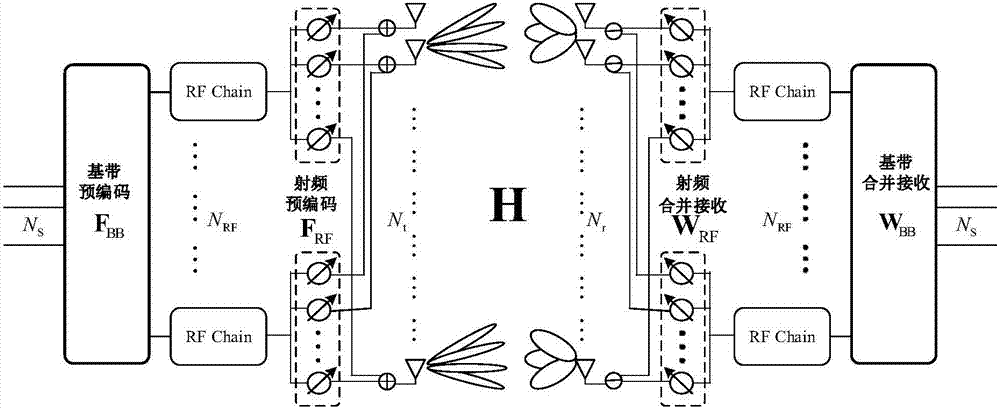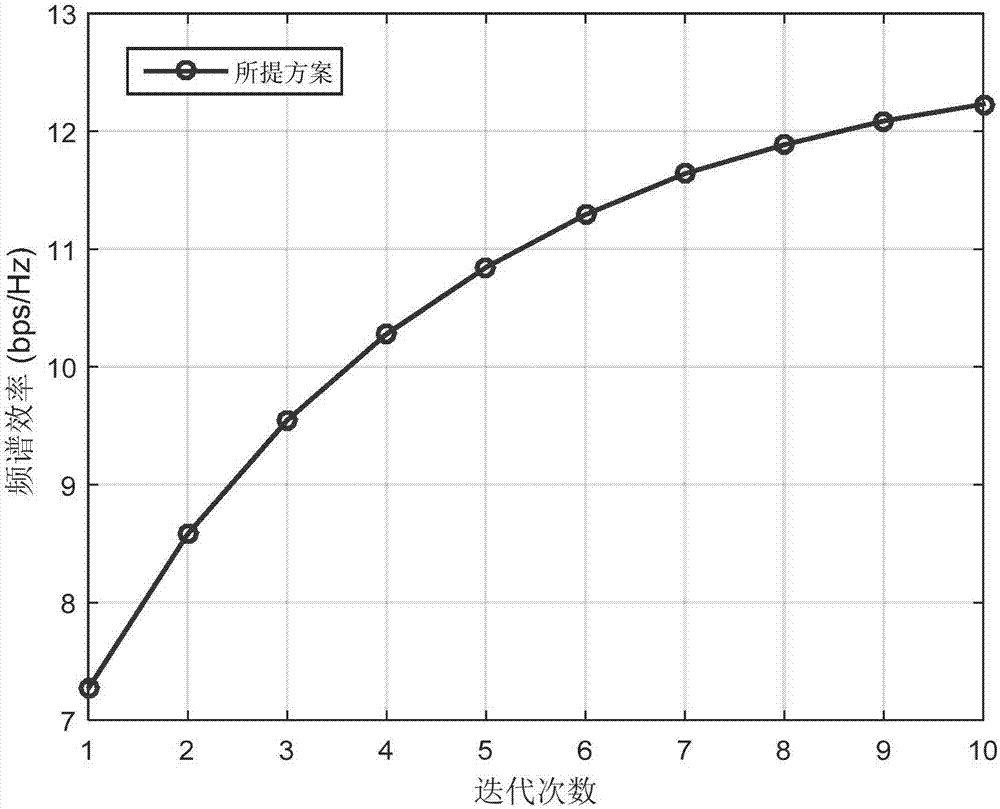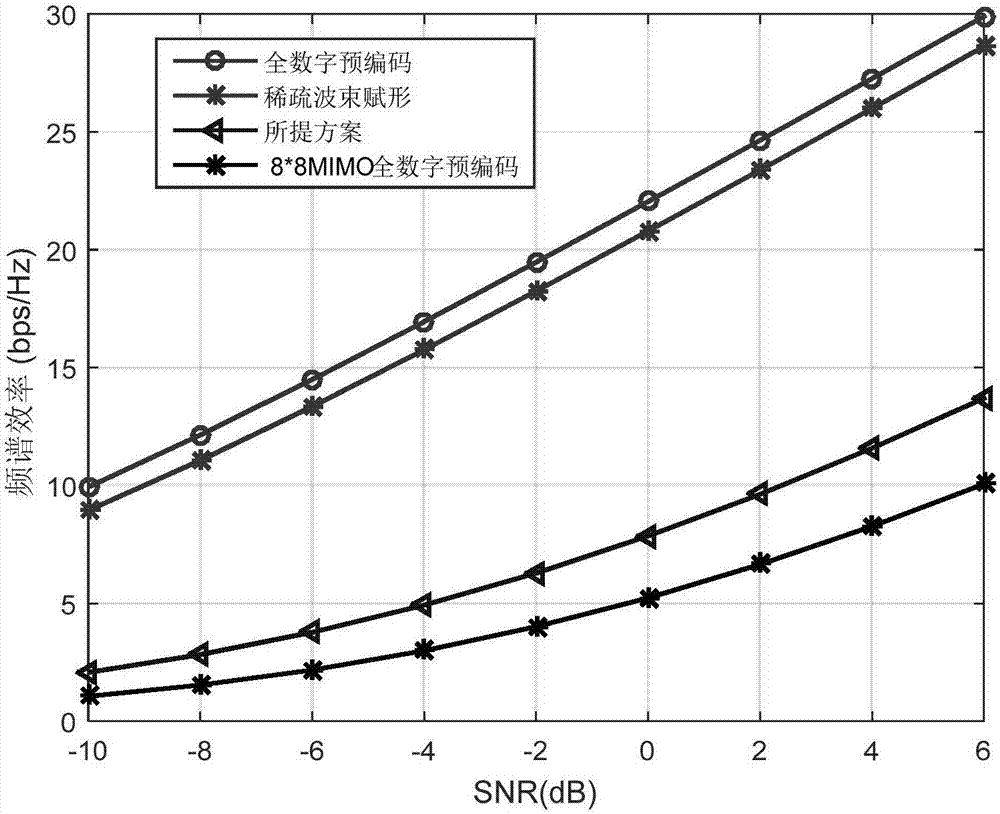# Hybrid beam forming iterative design method with low complexity

## A hybrid beam, low-complexity technology, applied in the field of low-complexity hybrid beamforming iterative design, can solve problems such as difficulty in obtaining accurate original channel state information, and achieve the effects of reducing signaling overhead and cost

Active Publication Date: 2017-09-05
XI AN JIAOTONG UNIV
7 Cites 25 Cited by

## AI-Extracted Technical Summary

### Problems solved by technology

Obtaining accurate raw channel stat...
View more

## Abstract

The invention discloses a hybrid beam forming iterative design method with low complexity. The method comprises the following steps: 1) analog beam forming design: first step, randomly selecting a vector from a DFT network, and initializing an analog beam forming matrix; second step, fixing the analog beam forming matrix and a receiving end equivalent channel, and then restoring the receiving end equivalent channel through the analog beam forming matrix; third step, fixing an analog pre-encoding matrix, updating an analog receiving matrix by a receiving end through the receiving end equivalent channel, and updating a feedback equivalent channel; fourth step, restoring a transmitting end equivalent channel by a transmitting end by using the equivalent channel; fifth step, fixing the analog receiving matrix, and updating the analog pre-encoding matrix through the transmitting end equivalent channel; repeating the second step to the fifth step until algorithm convergence; 2) digital beam forming design: first step, calculating the equivalent channel according to the determined analog beam forming matrix; and second step, calculating a digital beam forming matrix by using a singular value decomposition method, and performing power allocation by using an irrigation method.

Application Domain

Spatial transmit diversity

Technology Topic

Low complexityBeamforming +6

## Image

•••## Examples

• Experimental program(1)

### Example Embodiment

 The present invention will be described in further detail below in conjunction with the accompanying drawings:
 The main idea of ​​the low-complexity hybrid beamforming iterative design scheme proposed by the present invention is to divide the hybrid beamforming design process into two simulation stages. The first stage: iteratively design the analog precoding and analog combined receiving matrix at the transceiver end; the first stage: after determining the analog precoding and analog combined receiving matrix, design the digital precoding and digital combined receiving matrix in the digital domain according to the equivalent channel .
 The specific implementation plan is as follows:
 Consider a downlink millimeter wave massive MIMO system, the base station uses N t Antenna, Radio frequency transmission signal, users use N r Antenna, The radio frequency receiving signal, that is, both ends of the transceiver adopt a hybrid beamforming frame structure. F RF Is the analog precoding matrix at the radio frequency end of the base station, F BB Is the digital precoding matrix at the baseband. The present invention assumes that the millimeter wave channel is a narrowband slow fading channel. After the received signal is processed by the analog receiving combining matrix and the digital combining matrix, it can be expressed as

 Where W RF It is an analog merging matrix, which is the same as analog precoding and is implemented by a phase shifter network, so all its elements have the same amplitude, W BB Represents the digital combined receiving matrix. Represents additive white Gaussian noise, subject to a mean value of 0, and a variance of Gaussian distribution.
 From equation (1), the spectrum efficiency of the system can be expressed as:

 among them, Represents the covariance matrix of the noise at the receiving end.
 According to the above model, the objective of the present invention is to find the optimal hybrid precoding matrix and hybrid receiving matrix, so as to maximize the spectrum efficiency of the system. The problem can be described as a mathematical optimization problem as follows:

 among them, Is a feasible set of analog precoding, that is, a set of all elements with the same amplitude Matrix set, It is a feasible set of simulated combined reception, that is, a set of all elements with the same amplitude Matrix set.
 After decoupling the analog and digital domains, the achievable spectrum efficiency of the system can be expressed as

 Where Represents the covariance matrix of noise after the simulated merge matrix, It is for variable substitution of analog precoding and digital precoding de-power coupling.

 definition The goal of the above formula is to find a pair (W RF ,F RF ) To maximize the equivalent channel capacity, the above formula can be further expressed as

 From Jensen's inequality, the present invention can relax the optimization problem (6) as

 Based on the above theoretical derivation, the low-complexity hybrid beamforming iterative design scheme proposed by the present invention is as follows:
 1) Iterative design of analog precoding matrix and analog combined receiving matrix
 The first step, initialization: from the DFT matrix choose randomly Column as initial From the DFT matrix choose randomly Column as initial Let the number of iterations k=0;
 The second step, given with Receiver estimate equivalent channel make Then calculate
 The third step is to fix the analog precoding matrix Update the simulated merge receiving matrix, that is, Then use Update And feed it back to the sender;
 The fourth step, the sender uses the obtained equivalent channel Restore the equivalent channel of the sender, that is Then calculate
 The fifth step is to fix the analog combined receiving matrix Update the analog precoding matrix, that is,
 k←k+1; Repeat the first to fifth steps until the algorithm converges.
 2) Design of digital precoding matrix and digital combined receiving matrix
 The first step is to calculate the equivalent channel
 The second step, equivalent channel H eff Perform singular value decomposition to obtain the digital precoding matrix, namely Optimal digital precoding matrix Where N S Represents the number of data streams, the diagonal element of the diagonal matrix Λ represents the power allocated to each data stream, which can be obtained by the irrigation algorithm, and the corresponding power on the sth data stream can be expressed as ρ s , Which meets

 Where μ satisfies The optimal digital receiving combination matrix is ​​determined by the equivalent channel H eff The top N of the left singular matrix corresponding to the largest singular value S A column vector, namely
 Table 1 Comparison of the number of radio frequency links and signaling overhead required by different solutions.


## PUM## Description & Claims & Application Information

We can also present the details of the Description, Claims and Application information to help users get a comprehensive understanding of the technical details of the patent, such as background art, summary of invention, brief description of drawings, description of embodiments, and other original content. On the other hand, users can also determine the specific scope of protection of the technology through the list of claims; as well as understand the changes in the life cycle of the technology with the presentation of the patent timeline. Login to view more.

## Magnetically guided catheter

ActiveUS20100174177A1low costhigh performance
Owner:ST JUDE MEDICAL ATRIAL FIBRILLATION DIV

## Method for treating a solid material to make it hydrophobic, material obtained and uses

InactiveUS6342268B1simple and rapid methodlow-cost
Owner:BT3 TECH

## OLED apparatus having improved fault tolerance

ActiveUS20050174064A1reduce likelihoodlow cost
Owner:GLOBAL OLED TECH

## Material including graphene and an inorganic material and method of manufacturing the material

ActiveUS20110129675A1reduce defectlow cost
Owner:SAMSUNG ELECTRONICS CO LTD +1

## Preparing method of bamboo fiber winding composite tube

InactiveCN101571213Alow costImprove toughness
Owner:XINJIANG GUANGSHUI PIPE

• low cost

## Data transmission method and data transmission device for ultra-dense network

Owner:MEIZU TECH CO LTD

## Transmission instruction, transmission method and device for periodical detected reference signal

Owner:DATANG MOBILE COMM EQUIP CO LTD

## Random access method, user equipment and base station equipment

Owner:BEIJING SAMSUNG TELECOM R&D CENT +1

## Method and system for switching multi-input and multi-output modes of LTE system

Owner:COMBA TELECOM SYST CHINA LTD
Who we serve
• R&D Engineer
• R&D Manager
• IP Professional
Why Eureka